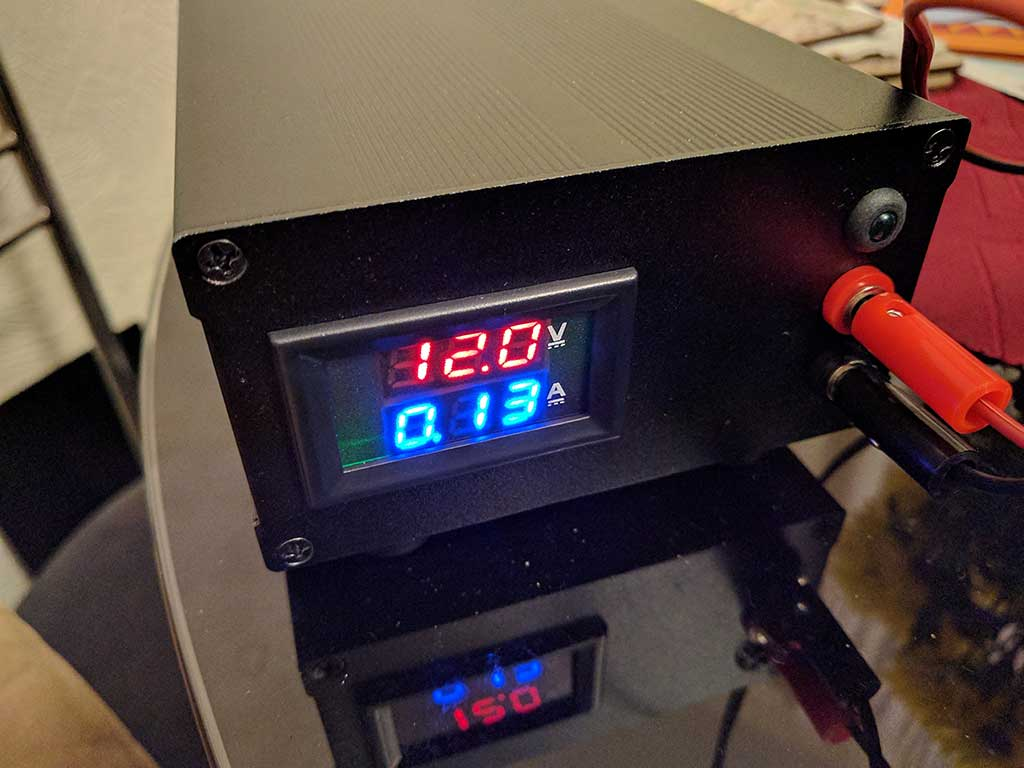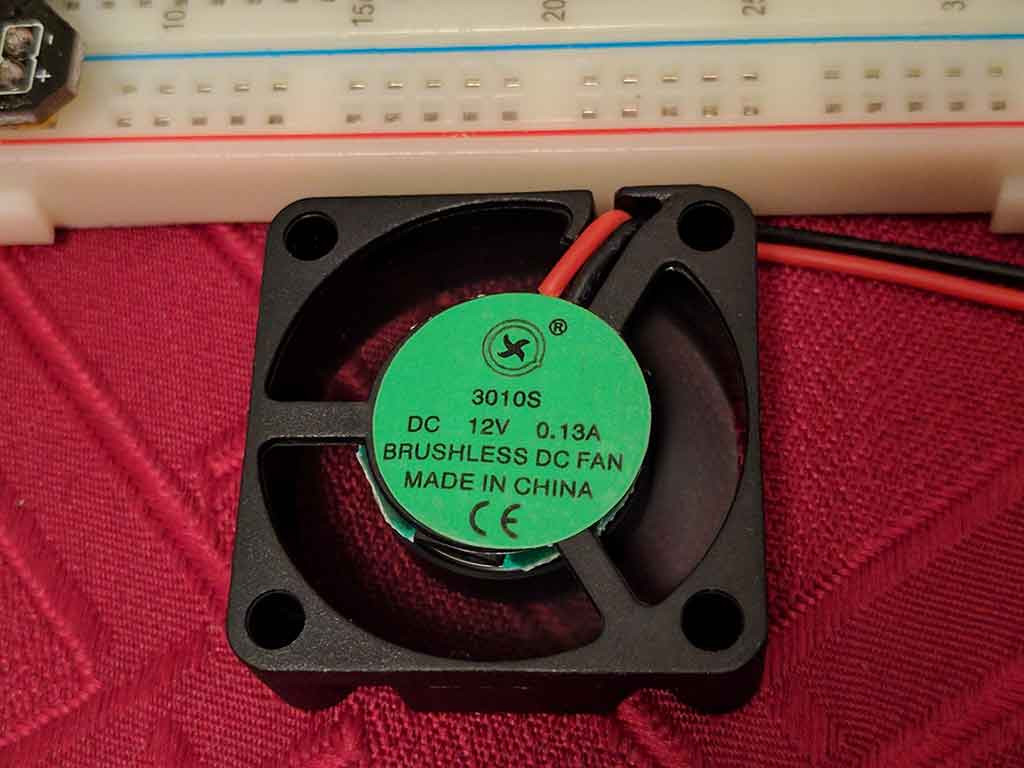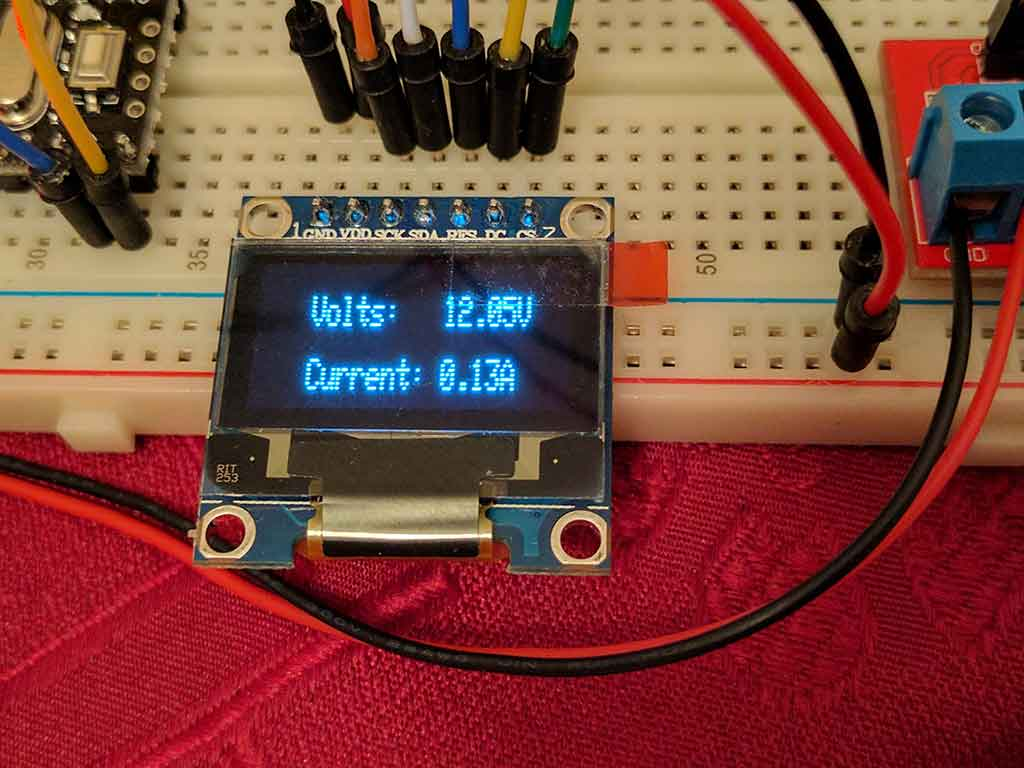# B4R Code SnippetReading and displaying voltage and current made easy with the Max471

SubName: Reading voltage and current and display the results onto an OLED display.
Description: Use the code below to display the voltage and current from load connected to a MAX471 module. The Max471 is a low cost precision high side current sense amplifiers with a maximum supply voltage of 36V. I personally would not push it that high, but putting 25V through it for a 5V Arduino or compatible device is more than adequate.

In the code below (4.91) and (1.15) were calibrated to match my multimetres readings, the Pro Mini was being powered by exactly 4.832V through the USB to Serial converter, for those of you that are interested in those sort of things.

The code below allows you to retrieve the readings from the Max471 and show the results on a display. I found that updating the results every 500 milliseconds was a nice update rate for me.

B4X:
``````'INPUT WARNING
'Using 5V Arduino, input voltage range 3VDC to 25VDC
'Using 3.3V Arduino/Wemos, input voltage range 3VDC to 16.5VDC
'Current range is 0A to 3A

'WIRE LEGEND for Max471 Vontage and Current board
'GND = GND
'VT = A0
'AT = A1

'WIRE LEGEND for 12864 7 pin OLED display
'VCC (VDD)= 3.3V
'GND = GND
'D0 (SCK) = D13
'D1 (SDA) = D11
'RST (RES) = D8
'DC = D9
'CS (SS) = D10

Sub Process_Globals
'These global variables will be declared once when the application starts.
'Public variables can be accessed from all modules.
Public Serial1 As Serial

Private V_Pin_A0, A_Pin_A1 As Pin
End Sub

Private Sub AppStart
Serial1.Initialize(115200)
Log("AppStart")

SSD.InitializeHSPI(9, 8, 10)
SSD.ClearDisplay
SSD.GFX.ConfigureText(1, SSD.WHITE, False)

End Sub

Dim Voltage, VTmp, Current, ATmp, AWV As Float 'AWV = Arduino Working Voltage 3.3V or 5V

AWV = 5 'Will either be 3.3 or 5 depending on your Arduino's Working Voltage
Voltage = VTmp * (AWV / 1023) * (4.91) 'Manually adjust to calibrate, default is (5).
Current = ATmp * (AWV / 1023) * (1.15) 'Manually adjust to calibrate, default is (1).

Log("Volts = ", NumberFormat(Voltage, 1, 2), ", Current = ", NumberFormat(Current, 1, 2))

SSD.ClearDisplay
SSD.GFX.SetCursor(23, 7)
SSD.GFX.DrawText(JoinStrings(Array As String("Volts:   ", Voltage, "V")))
SSD.GFX.SetCursor(23,21)
SSD.GFX.DrawText(JoinStrings(Array As String("Current: ", Current, "A")))
SSD.Display
End Sub``````

The detection feature of the Max471 is surprisingly quite stable, I thought that the results would be jumping all over the place, so calculating to 1 decimal place would basically be spot on.

Considering that this is a cheap \$1 module, I thought that it would be a lot worse.

AppStart
Volts = 12.05, Current = 0.14
Volts = 12.02, Current = 0.12
Volts = 12.00, Current = 0.13
Volts = 12.07, Current = 0.15
Volts = 12.07, Current = 0.12
Volts = 12.02, Current = 0.13
Volts = 12.02, Current = 0.12
Volts = 12.02, Current = 0.12
Volts = 12.07, Current = 0.12
Volts = 12.05, Current = 0.13
Volts = 12.05, Current = 0.11
Volts = 12.07, Current = 0.13
Volts = 12.07, Current = 0.13
Volts = 12.07, Current = 0.13
Volts = 12.07, Current = 0.12
Volts = 12.12, Current = 0.14
Volts = 12.02, Current = 0.13
Volts = 12.02, Current = 0.13
Volts = 12.02, Current = 0.13
Volts = 12.00, Current = 0.13
Volts = 12.02, Current = 0.12
Volts = 12.05, Current = 0.12
Volts = 12.05, Current = 0.11
Volts = 12.02, Current = 0.13
Volts = 12.00, Current = 0.12
Volts = 12.07, Current = 0.13
Volts = 12.05, Current = 0.12
Volts = 12.02, Current = 0.13
Volts = 12.00, Current = 0.13
Volts = 0.00, Current = 0.00

Tags: USB to Serial, Pro Mini, MAX471, Display, Voltage, Current

How it looks...

Powering the fan with my home made modular desktop power supply. I still need to add 2 x 10 turn potentiometers for volts and amps, but for this project I manually set the power output to 12v internally.I'm using a 12v fan as load on the Max471 voltage and current sensor module.I'm using an Arduino Pro Mini (middle), USB to Serial converter (top), Max471 voltage and current sensor (right) and 12864 OLED display (bottom).After calibrating the volts and current reading via the source code at 12V, I found that the readings were basically correct when measuring between 5VDC and 24VDC whilst using a dummy load. The readings also matched the readings on my desktop power supply and my 2 multimeters (one was connected in parallel to measure the voltage, the other was connected inline to measure the current).Enjoy...

Last edited:

Replies
2
Views
422
B4R Library rLegoinoBoost
Replies
1
Views
756
Replies
0
Views
2K
Replies
4
Views
889
Replies
5
Views
787